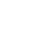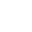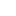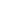# Prim’s – Minimum Spanning Tree (MST) |using Adjacency List and Priority Queue without decrease key in O(ElogV)

Earlier we have seen the implementation of prim’s algorithm using priority queue with decrease key and how decrease key has increased the time complexity. In this article we will improve the implementation(without decrease key) and reduce the complexity from O(EVlogV) to O(ElogV).

If you are reading prim’s algorithm for the first time then we recommend reading the following articles before continuing

Example:Why one more implementation:

First we saw implementation using adjacency matrix which was easy to implement but complexity was O(V2), then we discussed implementation using adjacency list with priority queue but complexity got worst  O(EVlogV). After that we discussed adjacency list with min-Heap and we got the best results with time complexity O(ElogV) but we implemented own min-heap with made the implantation but complex. In this article will achieve same time complexity O(ElogV) using priority queue.

How our earlier implementation using priority queue was not efficient

In our earlier implementation of prim’s algorithm using priority queue with decrease key function, the time complexity was on the higher side because of decrease key function. Since there is no easy way to implement the decrease key in priority queue we followed the expensive approach. In this article we will implement it without using decrease key.

Let’s first see the decrease key algorithm for priority queue.

• Iterate through all the nodes in priority queue.
• Find the node for which we want to decrease the key.
• Once find the node, remove it from the priority queue update it and add it again.

Improvements:

• Do not insert all the vertices in the beginning.
• Insert only vertices which are not in MST and has been visited through one of the vertex.
• Use Pair Class and use pair object as a priority queue node. (Please read about Pair class)

Complete Algorithm:

1. Create priority queue of size = no of vertices.
2. Will create pair object for each vertex with two information’s, vertex and key. (similar to heap node)
3. Override the Comparator of priority queue to sort them based on the key
4. Use mst[] to keep track of the vertices which are currently in MST.
5. Create key[] to keep track of key value for each vertex. , initialize all keys as MAX_VAL for the first vertex for which key will 0. (Start from first vertex).
6. Create a pair object for vertex 0 with key 0 and insert into priority queue.
7. while priority queue is not empty
1. Extract the min node from the priority queue, say it vertex u and add it to the MST, mst[u] = true.
2. Iterate through all the adjacent vertices of above vertex u and if adjacent vertex(say it’s v) and if v is not in MST and key of vertex v> u-v weight then create a pair object with vertex v and key = u-v weight and insert it into priority queue.
3. Update the key[v] = u-v
8. We will use Result object to store the result of each vertex. Result object will store two information’s.
1. First the parent vertex means from which vertex you can visit this vertex. Example if for vertex v, you have included edge u-v in mst[] then vertex u will be the parent vertex.
2. Second weight of edge u-v. If you add all these weights for all the vertices in mst[]  then you will get Minimum spanning tree weight.

See the animation below for more understanding.Time Complexity:

Total vertices: V, Total Edges : E

• O(logV) – to extract each vertex from queue. So for V vertices – O(VlogV)
• O(logV) – each time new pair object with new key value of a vertex and will be done for at most once for each edge. So for total E edge – O(ElogV)
• So over all complexity: O(VlogV) + O(ElogV) = O((E+V)logV) = O(ElogV)

Complete Code: <

Output:

```Minimum Spanning Tree:
Edge: 1 - 2 key: 1
Edge: 2 - 0 key: 3
Edge: 3 - 1 key: 2
Edge: 4 - 3 key: 2
Edge: 5 - 4 key: 6
Total minimum key: 14```

__________________________________________________
Top Companies Interview Questions..-

If you find anything incorrect or you feel that there is any better approach to solve the above problem, please write comment.
__________________________________________________